Normal view MARC view ISBD view

# Mathematics of quantization and quantum fields / Jan Dereziński, University of Warsaw, Poland, Christian Gérard, Universite de Paris-Sud, France.

Material type:TextPublisher: c2013Description: xii, 674 pages ; UKP 90.00 26 cm.ISBN: 9781107011113 (hbk.) :; 1107011116 (hbk.) :.DDC classification: 530.120151
Contents:
Machine generated contents note: Preface; 1. Vector spaces; 2. Operators in Hilbert spaces; 3. Tensor algebras; 4. Analysis in L2(Rd); 5. Measures; 6. Algebras; 7. Anti-symmetric calculus; 8. Canonical commutation relations; 9. CCR on Fock spaces; 10. Symplectic invariance of CCR in finite dimensions; 11. Symplectic invariance of the CCR on Fock spaces; 12. Canonical anti-commutation relations; 13. CAR on Fock spaces; 14. Orthogonal invariance of CAR algebras; 15. Clifford relations; 16. Orthogonal invariance of the CAR on Fock spaces; 17. Quasi-free states; 18. Dynamics of quantum fields; 19. Quantum fields on space-time; 20. Diagrammatics; 21. Euclidean approach for bosons; 22. Interacting bosonic fields; Subject index; Symbols index.
Summary: "Unifying a range of topics that are currently scattered throughout the literature, this book offers a unique and definitive review of mathematical aspects of quantization and quantum field theory. The authors present both basic and more advanced topics of quantum field theory in a mathematically consistent way, focusing on canonical commutation and anti-commutation relations. They begin with a discussion of the mathematical structures underlying free bosonic or fermionic fields, such as tensors, algebras, Fock spaces and CCR and CAR representations (including their symplectic and orthogonal invariance). Applications of these topics to physical problems are discussed in later chapters. Although most of the book is devoted to free quantum fields, it also contains an exposition of two important aspects of interacting fields: diagrammatics and the Euclidean approach to constructive quantum field theory. With its in-depth coverage, this text is essential reading for graduate students and researchers in departments of mathematics and physics"--
Item type Current location Call number Status Date due Barcode Item holds
Book Chennai Mathematical Institute
General Stacks
530.15 DER (Browse shelf) Available 9236
Total holds: 0
##### Browsing Chennai Mathematical Institute Shelves , Shelving location: General Stacks Close shelf browser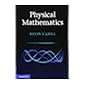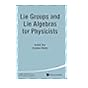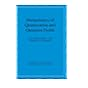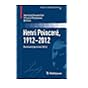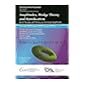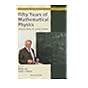530.15 CAH Physical mathematics / 530.15 DAS Lie groups and Lie algebras for physicists / TRiPS 14 530.15 DAS Integrable models / 530.15 DER Mathematics of quantization and quantum fields / 530.15 DUP Henri Poincaré, 1912-2012 : Poincaré Seminar 2012 / 530.15 EBR Amplitudes, Hodge theory and ramification : from periods and motives to Feynman amplitudes : 2014 Clay Institute Summer School : periods and motives : Feynman amplitudes in the 21st century, June 30-July 25, 2014, Instituto de Ciencias Matemáticas, Madrid, Spain / 530.15 FAD Fifty years of mathematical physics : selected works of Ludvig Faddeev /

Formerly CIP. Uk

Includes bibliographical references (pages 661-667) and indexes.

Machine generated contents note: Preface; 1. Vector spaces; 2. Operators in Hilbert spaces; 3. Tensor algebras; 4. Analysis in L2(Rd); 5. Measures; 6. Algebras; 7. Anti-symmetric calculus; 8. Canonical commutation relations; 9. CCR on Fock spaces; 10. Symplectic invariance of CCR in finite dimensions; 11. Symplectic invariance of the CCR on Fock spaces; 12. Canonical anti-commutation relations; 13. CAR on Fock spaces; 14. Orthogonal invariance of CAR algebras; 15. Clifford relations; 16. Orthogonal invariance of the CAR on Fock spaces; 17. Quasi-free states; 18. Dynamics of quantum fields; 19. Quantum fields on space-time; 20. Diagrammatics; 21. Euclidean approach for bosons; 22. Interacting bosonic fields; Subject index; Symbols index.

"Unifying a range of topics that are currently scattered throughout the literature, this book offers a unique and definitive review of mathematical aspects of quantization and quantum field theory. The authors present both basic and more advanced topics of quantum field theory in a mathematically consistent way, focusing on canonical commutation and anti-commutation relations. They begin with a discussion of the mathematical structures underlying free bosonic or fermionic fields, such as tensors, algebras, Fock spaces and CCR and CAR representations (including their symplectic and orthogonal invariance). Applications of these topics to physical problems are discussed in later chapters. Although most of the book is devoted to free quantum fields, it also contains an exposition of two important aspects of interacting fields: diagrammatics and the Euclidean approach to constructive quantum field theory. With its in-depth coverage, this text is essential reading for graduate students and researchers in departments of mathematics and physics"--